### Home > PC > Chapter 8 > Lesson 8.1.3 > Problem8-40

8-40.
1. Without using your calculator, find the following limits.Homework Help ✎

1.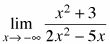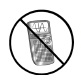2.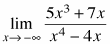3.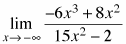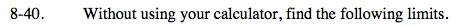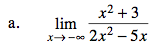Since the dominant term is in both the numerator and the denominator, the limit is

$\frac {1}{2}; \text{ (the ratio of their coefficients).}$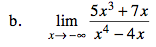Since the dominant term is in the denominator, the limit is 0.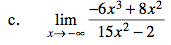Since the dominant term is in the numerator, the limit is +∞.
The negative input will be multiplied by itself 4 times.